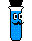## Stoichiometry Problem Solving

 Acid-Base Titration 1 Titration of a base with an acid. Empirical Formula 1 Calculation of the empirical formula from mass data. Empirical Formula 2 Calculation of the empirical formula from percent composition data. Molecular Formula 1 Calculation of the molecular formula from an empirical formula and the molecular mass. Molecular Formula 2 Calculation of the molecular formula from percent composition and the molecular mass. Percent Mass Calculation of percent mass from the molecular formula.

These pages present some stoichiometry problems and strategies for solving them. The pages recommend a problem solving strategy then show you how to work through each step of the problem.

As you work through the problems, you will notice that:

• The current step is displayed in bold.
• Information used in the current step is highlighted in red.
• The frames on the page are resizable so that you can display the information you would like to display.

Grab your pencil and paper and use the worked steps to help you if you get stuck. After successfully completing a problem with the computer, try choosing a related homework problem and applying the strategy to it.

To begin, select a problem from above.General Chemistry Help HomepageProblem Solving